# NCERT Solutions for Chapter 6 Integers Class 6 Maths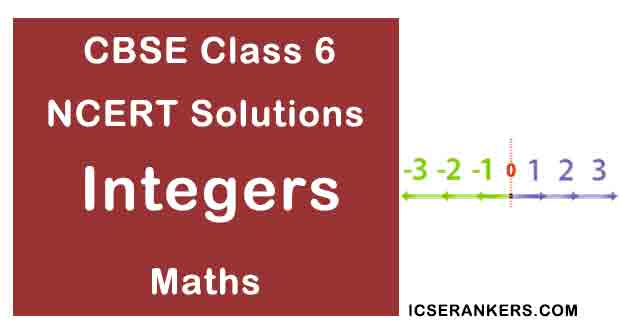Chapter Name Integers NCERT Solutions Class CBSE Class 6 Textbook Name Maths Related Readings NCERT Solutions for Class 6NCERT Solutions for Class 6 Maths Summary of Integers

Exercise 6.1

Question 1: Write opposite of the following:

(a) Increase in weight

(b) 30 km north

(c) 326 BC

(d) Loss of ₹700

(e) 100 m above sea level

Solution

(a) Decrease in weight

(b) 30 km south

(d) Profit of ₹700

(e) 100 m below sea level

Question 2: Represent the following numbers as integers with appropriate signs.

(a) An aeroplane is flying at a height two thousand meters above the ground.

(b) A submarine is moving at a depth eight thousand meters below the sea level.

(c) A deposit of rupees two hundred.

(d) Withdrawal of rupees seven hundred.

Solution

(a) (+) 200 meters

(b) (–) 800 meters

(c) (+) 200 Rupees

(d) (–) 700 Rupees

Question 3: Represent the following numbers on number line:

(a) +5

(b) –10

(c) +8

(d) –1

(e) –6

Solution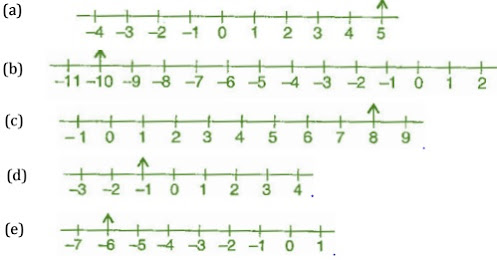Question 4: Following figure is a vertical number line, representing integers. Observe it and locate the following points: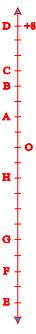(a) If point D is +8 then which point is –8?

(b)Is point G a negative integer or a positive integer?

(c) Write integers for points B and E.

(d) Which point marked on this number line has the least value?

(e) Arrange all the points in decreasing order of values

Solution.

(a) F

(b) Negative

(c) B = (+) 4; E = (–) 10

(d) E

(e) D, C, B, A, O, H, G, F, E

Question 5: (a) Following is the list of temperatures of five places in India, on a particular day of the year.

Place                  Temperature

Siachin              10oC below 0oC      ________

Shimla               2oC below 0oC        ________

Delhi                  20oC above 0oC      ________

Srinagar            5oC below 0oC        ________

(a) Write the temperature of these places in the form of integers in the blank column.

(b) Following is the number line representing the temperature in degree Celsius.Plot the name of the city against its temperature.

(c) Which is the coolest place?

(d) Write the names of the place where temperature are above 10oC.

Solution

(a)

Place           Temperature

Siachin           (–) 10oC

Shimla            (–) 2oC

Delhi                (+) 20oC

Srinagar           (–) 5oC

(b) Number line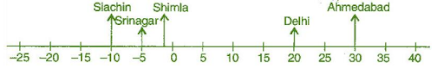(c) Siachin

Question 6: In each of the following pairs, which number is to the right of the other on the number line?

(a) 2, 9

(b) –3, –8

(c) 0, –1

(d) –11, 10

(e) –6, 6

(f) 1, –100

Solution

(a) 9 is right to 2

(b) –3 is right to –8

(c) 0 is right to –1

(d) 10 is right to –11

(e) 6 is right to –6

(f) 1 is right to –100

Question 7: Write all the integers between the given pairs (write them in the increasing order):

(a) 0 and –7

(b) –4 and 4

(c) –8 and –15

(d) –30 and –23

Solution

(a) –6, –5, –4, –3, –2, –1

(b) –3, –2, –1, 0, 1, 2, 3

(c) –14, –13, –12, –11, –10, –9

(d) –29, –28, –27, –26, –25, –24

Question 8: (a) Write four negative integers greater than –20.

(b) Write four negative integers less than –10.

Solution

(a) –19, –18, –17, –16

(b) –11, –12, –13, –14

Question 9: For the following statements write True (T) or False (F). If the statement is false, correctthe statement:

(a) –8 is to the right of –10 on a number line.

(b) –100 is the right of –50 on a number line.

(c) Smallest negative integer is –1.

(d) –26 is larger than –25.

Solution

(a) True

(b) False

(c) False

(d) False

Question 10: Draw a number line and answer the following:

(a) Draw a number line will we reach if we move 4 numbers to the right of –2.

(b) Which number will we reach if we move 5 numbers to the left of 1.

(c) If we are at –8 on the number line, in which direction should we move to reach –13?

(d)If we are at –6 on the number line, in which direction should we move to reach –1?

Solution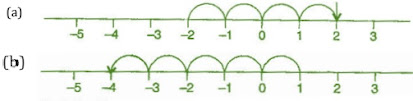(c) On left side

(d) On right side

Exercise 6.2

Question 1: Using the number line write the integer which is:

(a) 3 more than 5

(b) 5 more than –5

(c) 6 less than 2

(d) 3 less than –2

Solution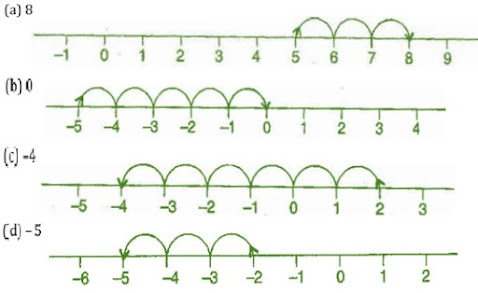Question 2: Use number line and add the following integers:

(a) 9 + (–6)

(b) 5 + (–11)

(c) (–1) + (–7)

(d) (–5) + 10

(e) (–1) + (–2) + (–3)

(f) (–2) + 8 + (–4)

Solution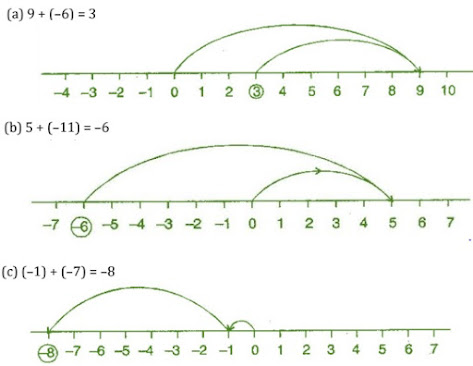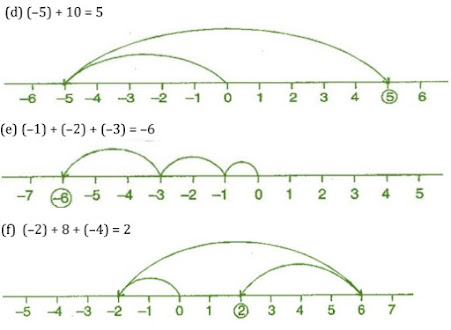Question 3: Add without using number line:

(a) 11 + (–7)

(b) (–13) + (+18)

(c) (–10) + (+19)

(d) (–250) + (+150)

(e) (–380) + (–270)

(f) (–217) + (–100)

Solution

(a) 11 + (–7) = 11 – 7 = 4

(b) (–13) + 18 = 5

(c) (–10) + (+19) = –10 + 19 = 9

(d) (–250) + (+150) = –250 + 150 = –100

(e) (–380) + (–270) = –380 – 270 = –650

(f) (–217) + (–100) = –217 v 100 = –317

Question 4: Find the sum of:

(a) 137 and –354

(b) –52 and 52

(c) –213, 39 and 192

(d) –50, –200 and 300

Solution

(a) 137 + (–354) = 137 – 354 = –217

(b) (–52) + 52 = 0

(c) (–312) + 39 + 192 = –312 + 231 = –81

(d) (–50) + (–200) + 300 = –50 – 200 + 300 = –250 + 300 = 50

Question 5: Find the value of:

(a) (–7) + (–9) + 4 + 16

(b) 37 + (–2) + (–65) + (–8)

Solution

(a) (–7) + (–9) + 4 + 16

= –7 – 9 + 4 + 16

= –16 + 20

= 4

(b) 37 + (–2) + (–65) + (–8)

= 37 – 2 – 65 – 8

= 37 – 75

= –38

Exercise 6.3

Question 1: Subtract:

(a) 35 – (20)

(b) 72 – (90)

(c) (–15) – (–18)

(d) (–20) – (13)

(e) 23 – (–12)

(f) (–32) – (–40)

Solution

(a) 35 – 20 = 15

(b) 72 – 90 = –18

(c) (–15) – (–18) = –15 + 18 = 3

(d) –20 – (13) = –20 – 13 = –33

(e) 23 – (–12) = 23 + 12 = 35

(f) (–32) – (–40) = –32 + 40 = 8

Question 2: Fill in the blanks with >, < or = sign:

(a) (–3) + (–6) ____ (–3) – (–6)

(b)(–21) – (–10) ____ (–31) + (–11)

(c) 45 – (–11) ____ 57 + (–4)

(d)(–25) – (–42) ____ (–42) – (–25)

Solution

(a) (–3) + (–6)   <   (–3) – (–6)

(b) (–21) – (–10)   >  (–31) + (–11)

(c) 45 – (–11)   >  57 + (–4)

(d) (–25) – (–42)    >  (–42) – (–25)

Question 3: Fill in the blanks:

(a) (–8) + ____ = 0

(b) 13 + ____ = 0

(c) 12 + (–12) = ____

(d)(–4) + ____ = –12

(e) ____ – 15 = –10

Solution

(a) (–8) + 8 = 0

(b) 13 + (–13) = 0

(c) 12 + (–12) = 0

(d) (–4) + (–8) = –12

(e) 5 – 15 = –10

Question 4: Find:

(a) (–7) – 8 – (–25)

(b)(–13) + 32 – 8 – 1

(c) (–7) + (–8) + (–90)

(d) 50 – (–40) – (–2)

Solution

(a) (–7) – 8 – (–25)

= –7 – 8 + 25

= –15 + 25

= 10

(b) (–13) + 32 – 8 – 1

= –13 + 32 – 8 – 1

= 32 – 22

= 10

(c) (–7) + (–8) + (–90)

= –7 – 8 – 90

= –105

(d) 50 – (–40) – (–2)

= 50 + 40 + 2

= 92